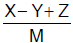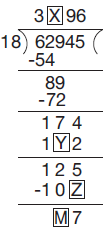# User ForumSubject :IMO    Class : Class 4

Find the value of.A3
B4
C5
D6

## Ans 1:

Class : Class 4
x = 4, y =6, z = 8, M = 1 therefore answer is 6

## Ans 2:

Class : Class 4
X = 4,y=6,z=8 and m=1 . Therefore the answer is B (6)

Class : Class 4
Ans-D